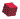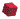Part 2:  Humanoid animation (HAnim) motion data animation

5 HAnim motion data animation using interpolators#5.1 General

## 5.1.1 Overview

This clause describes how interpolators may be used to produce motion data animation.

## 5.1.2 Topics

Table 5.1 lists the major topics in this clause.

Table 5.1 — Topics

#5.2 Definition of motion data animation using interpolators

## 5.2.1 Overview

This subclause specifies mapping motion data parameter values, typically obtained from motion-capture devices, and using these values to create interpolators for position and orientation. Generally, the values consist of translational and rotational channel values corresponding to all joints of a humanoid figure. For each frame, all transformation parameter values from motion data can be defined using interpolators.

An interpolator typically has a set of time-interval keys, with corresponding values at these keys, called key values. For use with HAnim motion animation the keys represent time, and are usually, but not necessarily, uniformly spaced over the total time period of the animation. The key values for HAnim motion animation may be position or orientation, depending on where they get applied.

## 5.2.2 Time data requirements

Motion data, typically obtained from motion capture, includes the number of frames of data, and the time per frame, in seconds. To specify the key array of an interpolator, the total duration of the animation shall be calculated using the following formula:

`    animationDuration = (frameCount - 1) × timePerFrame`

EXAMPLE  Suppose that motion-capture data starts with the following values:

```    Frames: 482
Frame Time: 0.016667
```

Frames denotes the total number of captured frames, and Frame Time denotes duration taken for displaying an individual frame.

If these units are seconds, the Frame Time matches 60 frames per second. The first entry in the time array is at time zero. Therefore, there are (482 - 1) = 481 intervals between frames in total. Thus, total duration = (number of intervals) * (frame time) = (481 * 0.016667) = (481 / 60) = 8.01667 seconds. Using this computation, the derived frame arrays are then driven by key values at appropriate frame-time increments.

## 5.2.3 Specification of key and key value arrays for interpolators

The key array is composed of time values, normally uniformly spread over the interval from zero to the end of the animation. This may be the actual animation duration, or it may be normalized to an end time of 1.0, if the total duration is used separately to scale the time values. The key array normally has the same values in all of the interpolators for an animation.

EXAMPLE 1 Normalizing the time values allows subsequent variation of the speed of the animation by simply changing the scaling factor, rather than generating a new set of interpolators.

EXAMPLE 2 Suppose that an interpolator key is a fraction from 0.0 to 1.0. Take the total time interval of a motion-capture file, e.g. 8.01667 seconds, divided by the number of intervals, e.g. 481. The resulting key array consists of fractions incrementing by (1.0 / 481). The result is:

`    key = "0  1/481  2/481  3/481  ...  1"`

Next, motion-capture parameter values for all channels are transferred to the key value arrays in the interpolators. Usually, motion capture parameter values are obtained frame by frame. For each frame, all motion-capture parameter values are obtained according to the joint hierarchy of the motion-capture figure.

A single position interpolator shall be specified, controlling the position of the HAnim figure through the course of the animation. Each key value has X, Y, and Z components. These values shall be applied to the translation field of the `"humanoid_root"` Joint object of the HAnim figure.

An orientation interpolator shall be specified for each joint in the HAnim figure that is to be animated. Each set of three rotation values in the motion data shall be used to calculate joint rotation angles. These joint rotation angle values are applied to the orientation field of the appropriate Joint object in the HAnim figure.

The type of the motion rotation angle data depends on the source of the data. In particular, the order of rotational values may differ. For example, in a motion-capture file, the order of angle data may be Zrotation, Xrotation, Yrotation, while an HAnim viewer may use the order of axes, x, y, z. The motion data angles, often called Euler angles, provide a value triplet (e.g. Zrotation, Xrotation, Yrotation) that shall be converted to a joint rotation axis angle quadruplet value (x y z angle), ensuring that the axis values are normalized to have a vector length of 1, and the angle value is given in radians.

## 5.2.4 Angle conversion procedure

A procedure for converting a motion-capture angle from an Euler angle representation in degrees to a joint axis angle in radians is as follows:

1. Choose the largest rotation angle among the three Euler angles.
2. Define the axis value as 1 for the rotation axis with the largest rotation angle for the quadruple.
3. Convert the largest angle in degrees into an angle in radians, which will be w for the quadruple.
4. The remaining axis values are calculated from the proportion of the rotation angle against the largest rotation angle.

EXAMPLE 1  Consider Euler angles x=90, y=45, z=-180. Using the procedure, the joint axis angle representation values are x=0.5, y=0.25, z=-1, w=3.14.

EXAMPLE 2  Consider Euler angles x=30, y=30, z=30. Using the procedure, the joint axis angle representation values are x=1, y=1, z=1, w=3.14/6.

An example implementation of this algorithm is shown below.

```    void HAnim::ConvertEulerToJointRotation(float& x, float& y, float& z, float& w)
{
#define piover180 0.01745329252f

float fAbsoluteX = abs(x);
float fAbsoluteY = abs(y);
float fAbsoluteZ = abs(z);

float fMaxAngle = 0;

if(fAbsoluteX > fMaxAngle) fMaxAngle = fAbsoluteX;
if(fAbsoluteY > fMaxAngle) fMaxAngle = fAbsoluteY;
if(fAbsoluteZ > fMaxAngle) fMaxAngle = fAbsoluteZ;

float fRatio = 0.0f; // avoid divide-by-zero problems
if(fMaxAngle > 0.0001f) fRatio = 1.0f / fMaxAngle;

x = x * fRatio;
y = y * fRatio;
z = z * fRatio;

w = fMaxAngle * π / 180;
w = abs(w);
}
```

## 5.2.5 Example of interpolator specification

Below is an example of a motion section (excluding the joint hierarchy section) from a motion-capture file:

```    Frame 0:
1.662 31.427 60.304
-1.249 -4.859 -3.582
4.463  1.354  0.075
-13.732  3.052  3.999
95.677  1.705 -1.512
5.541 -3.491  0.339
1.259 -3.022  1.790
6.765  2.405 -4.446
-91.027 -7.187  4.910
-3.633  0.867  0.043
-2.879  0.120 -5.688
-1.132 -1.858  0.809
-2.969 -8.472  1.461
-1.304  3.919 -2.045
1.054  9.006 -0.191
2.695 -1.341 -0.615
0.361  4.452  4.756
0.484  8.095  0.193
-6.340 -0.815  1.224
Frame 1:
11.659 31.427 60.307
-1.268 -4.835 -3.588
4.487  1.352  0.080
-13.802  3.059  3.999
95.651  1.737 -1.609
5.541 -3.521  0.340
1.298 -3.030  1.974
6.795  2.410 -4.418
-90.999 -7.145  4.917
-3.633  0.825  0.043
-2.862  0.151 -5.736
-1.141 -1.863  0.806
-2.988 -8.482  1.455
-1.283  3.890 -2.157
1.055  9.042 -0.193
2.781 -1.350 -0.638
0.381  4.430  4.692
0.484  8.102  0.194
-6.266 -0.819  1.206
```

In the example above, for each frame, one positional value (x, y, z) and a series of rotational values (x, y, z) are included. These positional and rotational parameter values are converted to key and key value arrays for position and orientation interpolators respectively.

The keyValue array for the PositionInterpolator is obtained from the first positional value for each motion-capture frame. The keyValue array for the OrientationInterpolator for each joint is obtained by the motion data rotation angle to joint axis angle conversion from the corresponding three values at each frame for each corresponding joint. The example below illustrates how the motion-capture data above can be converted to an X3D representation using Interpolator nodes.

```    <PositionInterpolator  DEF='HumanoidRootTransInterp'
key='0 1/481 2/481 3/481 ...'
keyValue='1.662 31.427 60.304,
1.659 31.427 60.307,
...' />
<OrientationInterpolator DEF='HumanoidRootRotInter'
key='0 1/481 2/481 3/481 ...'
keyValue='f(-1.249 -4.859 -3.582),
f(-1.268 -4.835 -3.588),
...' />
<OrientationInterpolator DEF='sacroiliacRotInterpo'
key='0 1/481 2/481 3/481 ...'
keyValue='f(4.463 1.354 0.075),
f(4.487 1.352 0.080),
... ' />
<OrientationInterpolator DEF='l_hipRotInterpolator'
key='0 1/481 2/481 3/481 ...'
keyValue='f(-13.732 3.052 3.999),
f(-13.802  3.059 3.999),
...' />
```

Conversion of motion data rotation angles to joint rotation axis angles is done by defining the function f(phi,theta,psi) for converting a single motion-capture angle triplet into a single joint rotation angle value. The function f(phi, theta, psi) represents the conversion HAnim:ConvertEulerToJointRotation detailed in 5.2.4 Example conversion procedure above.

#5.3 Comparison of different LOA motion data animation using interpolators

HAnim motion data animation using interpolators can be specified at different levels. The animation data differs in the number of orientation interpolators, depending on the level of articulation. A single position interpolator may be used for a motion data animation sequence. Table 5.2 shows a comparison of motion data animation using interpolators at different LOAs. Frame number is the number of total frames. Frame time is the same for all LOAs because LOA is not related to the time interval for displaying a frame.

Table 5.2 — Comparison of motion data animation using interpolators at different LOAs

Comparison item LOA 0 LOA 1 LOA 2 LOA 3 LOA 4
Difference of Frame number (number of frames) NO NO NO NO NO
Difference of Frame time NO NO NO NO NO
Number of TimeSensor 1 18 + 1 71 + 1 94 + 1 146 + 1
Number of PositionInterpolator 1 1 1 1 1
Number of OrientationInterpolator 1 18 71 94 146
Number of key in PositionInterpolator Frame number Frame number Frame number Frame number Frame number
Number of key value in PositionInterpolator Frame number Frame number Frame number Frame number Frame number
Number of key in OrientationInterpolator Frame number Frame number Frame number Frame number Frame number
Number of key value in OrientationInterpolator Frame number Frame number Frame number Frame number Frame number
ROUTE 2 × 1 2 × 19 2 × 72 2 × 94 2 × 146

#5.4 Excerpted example of interpolator animation

An excerpted example of motion data animation using interpolators is shown below. It uses the X3D representation. A full example is presented in Annex C.

This example also shows the connections, known as ROUTEs in X3D, that link the outputs of the interpolators to the joints of the HAnim figure. Also shown are the time control connections that are input to the interpolators.

```    <?xml version="1.0" encoding="UTF-8"?> <!DOCTYPE X3D PUBLIC "ISO//Web3D//DTD X3D 3.3//EN"
"http://www.web3d.org/specifications/x3d-3.3.dtd" >

<X3D profile="Immersive" version="3.3" xmlns:xsd="http://www.w3.org/2001/XMLSchema-instance"
xsd:noNamespaceSchemaLocation="http://www.web3d.org/specifications/x3d-3.3.xsd" >
<Scene>
<Viewpoint centerOfRotation="0 1 0" description="KoreanCharacter01Jin" position="0 1 3" />

<HAnimHumanoid DEF="hanim_HAnim" info="humanoidVersion=2.2" name="HAnim" scale="0.0225 0.0225 0.0225" version="2.2" >

<HAnimJoint DEF="hanim_humanoid_root" center="0.000000 30.530001 -0.707600" name="humanoid_root" containerField="skeleton" >
<HAnimSegment DEF="hanim_sacrum" name="sacrum" >
<Transform translation="0.00000 30.53000 -0.70760" >
<Shape>
<Appearance>
<Material diffuseColor ="0.58800 0.58800 0.58800" />
<ImageTexture  DEF="KoreanCharacter01JinTextureAtlas" url ="Jin.png" />
</Appearance>
<IndexedFaceSet creaseAngle ="3.14159" coordIndex ="0, 1, 2, -1, ... "
texCoordIndex ="0, 1, 2, -1, ... " >
<Coordinate point="0.0000 10.7900 0.1424, 0.0000 ... " />
<TextureCoordinate  point="0.6211 0.5754,0.7851 0.5720, ... " />
</IndexedFaceSet >
</Shape>
</Transform>
</HAnimSegment>

...

</HAnimJoint>
</HAnimHumanoid>

<Group DEF="197_aAnimation" >
<TimeSensor  DEF="197_aTimer" cycleInterval="8.033493996" enabled="true" loop="true" />
<PositionInterpolator DEF="197_a_HumanoidRoot" key="0.00000 0.00207 0.00414 ... " keyValue ="2.26997 42.92323 82.36365 ... " />
<OrientationInterpolator DEF="197_a_sacroiliac" key="0.00000 0.00207 0.00414 ... " keyValue ="-1.00000 -0.73718 -0.25704 ... " />
<OrientationInterpolator DEF="197_a_l_shoulder" key="0.00000 0.00207 0.00414 ... " keyValue ="0.30338 0.01680 1.00000 ... " />

...

<ROUTE fromField="fraction_changed" fromNode="197_aTimer" toField="set_fraction" toNode="197_a_HumanoidRoot" />
<ROUTE fromField="fraction_changed" fromNode="197_aTimer" toField="set_fraction" toNode="197_a_sacroiliac" />
<ROUTE fromField="fraction_changed" fromNode="197_aTimer" toField="set_fraction" toNode="197_a_l_shoulder" />

...

<ROUTE fromField="value_changed" fromNode="197_a_HumanoidRoot" toField="translation" toNode="hanim_humanoid_root" />
<ROUTE fromField="value_changed" fromNode="197_a_sacroiliac" toField="rotation" toNode="hanim_sacroiliac" />
<ROUTE fromField="value_changed" fromNode="197_a_l_shoulder" toField="rotation" toNode="hanim_l_shoulder" />

...

</Group>
</Scene>
</X3D>
```